# Aim of the Unit

This Unit aims to understand the graphical device and draw scatterplots.

# Introduction

This tutorial will show how to draw plots like this: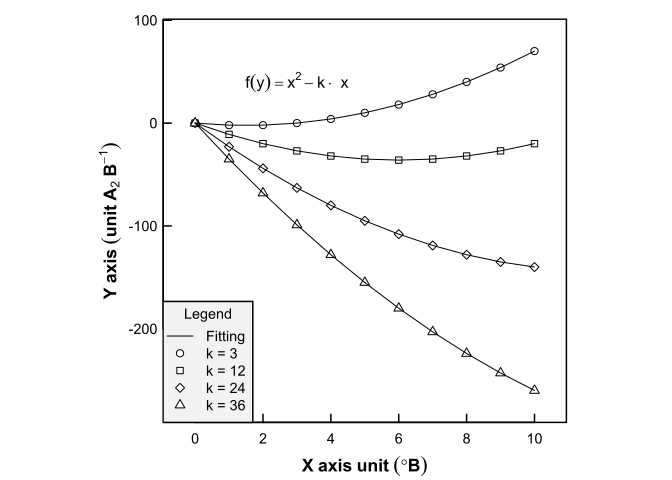What is needed to run this tutorial is the following dataset:

x <- seq(from = 0, to = 10, by = 1)
y1 <- x^2 - 3*x
y2 <- x^2 - 12*x
y3 <- x^2 - 24*x
y4 <- x^2 - 36*x
Y<-cbind(y1, y2, y3, y4)

The x-axis is the indipendent variable:

##    0  1  2  3  4  5  6  7  8  9 10

whereas, the y-axis is composed by four variables:

##       y1  y2   y3   y4
##  [1,]  0   0    0    0
##  [2,] -2 -11  -23  -35
##  [3,] -2 -20  -44  -68
##  [4,]  0 -27  -63  -99
##  [5,]  4 -32  -80 -128
##  [6,] 10 -35  -95 -155
##  [7,] 18 -36 -108 -180
##  [8,] 28 -35 -119 -203
##  [9,] 40 -32 -128 -224
## [10,] 54 -27 -135 -243
## [11,] 70 -20 -140 -260

# Overall strategy

To draw a high-quality figure you need to set:

1. Figure size
2. Margins
3. Box plot
4. Points
5. Lines
6. Axis
7. Lables
8. Thicks
9. Titles
10. Legend
11. Text expressions
12. Save

## Margins and figure size

R is able to manage complex scientific figures like scatterplot, box-plot or time series. As a first step, a few graphical parameters should be set by the function par().

The function par() should be called at the beginning of your script. With par(), you can define a number of graphical parameters. These are defined globally. Some of the most frequently used graphical parameters are used for two reasons:

1. Setting dimensions
2. Setting margins
Setting Function Description
Dimension pty = "s" Set a squared plot
fin = c(3.35, 3.35) Set the dimension of the figure (in inches)
Margin oma = c(3,4,1,1) Set the outer margin (in lines)
mar = c(3,4,1,1) Set the inner margin (in lines)
mgp = c(0,0.3,0) Set the margin for the axis title, axis labels and axis line

Such parameters should be defined with a synthax like this:

par(pty   = "s",
fin   = c(3.35,3.35),
oma   = rep(0,4),
mar   = c(3,4,1,1),
mgp   = c(0, 0.3, 0)
)

A detailed explaination of the meaning of each graphical parameters is offere in the help file of R. Just type ?par() on your R Console.

In the following sections, two of the most important graphical parameters will be described, respectively:

1. Dimensions
2. Margins

### Set figure size

The first graphical parameter to set is the dimension of the resulting figure. The size of the current graphical region is given by typing in the R Console the following code:

dev.size("in")
##  7 5

To set new dimension of the resulting graph, use the function par(fin = c("width", "height")):

par(fin   = c(3.35,3.35))    # THIS FIXES THE DIMENSION OF THE PLOT

Often, the dimension of the plot should have a width of half column of the paper. Typically, a paper has a width of 170 mm (or 6.7 inches). This means that the dimension of a one-column graph should not exceed 85 mm (or 3.35 inches).

### Margins

Among the graphcal parameters defined above, the most important are those that set the margins of the plot. Two graphical functions used for this purpose are the following:

Function Description
oma() Set the size of the outer margins in lines of text.
mar() Set the number of lines of margin on the four sides of the plot.

To better understand the meaning of these two functions, look at the following R graphical model: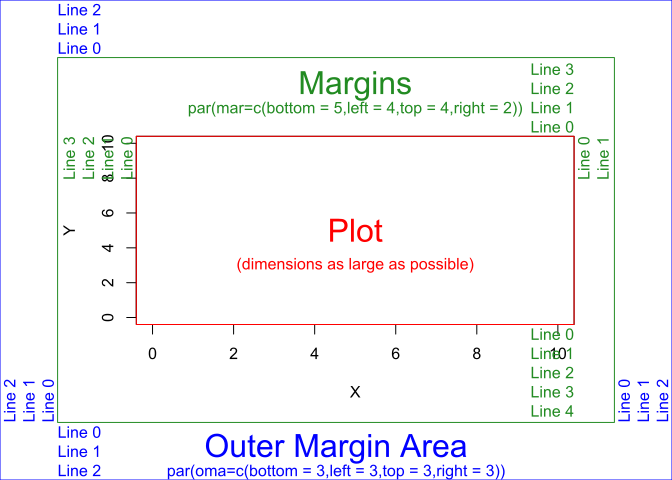To examplify the use of oma() and mar() functions, check out this code:

par(oma   = c(2,2,2,2) + 0.1) # Set 3 lines of space between the graphical device and the figure
par(mar   = c(3,3,3,3) + 0.1) # Set space between the figure and the plot
plot(x,y1)                    # Simple plot function
box(which = "plot" , lwd = 2) # Draw a box around the plot
box(which = "inner", lwd = 2) # Draw a box around the figure
box(which = "outer", lwd = 2) # Draw a plot around the device

that gives: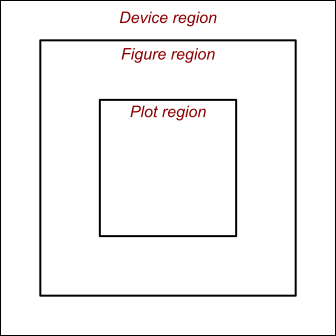Often, there is no need to account for outer margin. Thus, just set:

par(oma   = c(0,0,0,0)) # Set 3 lines of space between the graphical device and the figure  

The margin around the plot region should be enough to account for the axis title and tick labels. Gneral setting values are the following:

par(mar   = c(3,3,0,0) + 0.2) # Set space between the figure and the plot

These settings are invisible. Their effect will become visible later, when you will add to the plot other graphical elements, such as axis, box and lines. Now, red lines have been added in the following plot just to the the effect of oma and mar parameters: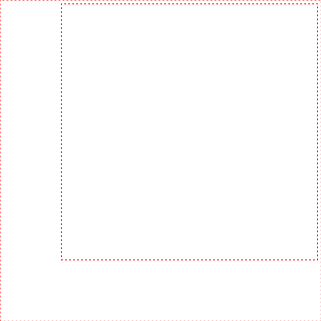The two red boxes are just useful to observe the effect of the oma() and mar() parameters.

## Second step: draw an empty plot

The next step is to initiate the grahical region. This is possible by drawing an empty plot with the function plot(type = "n"):

plot(0:10,0:10, # X AND Y DATA
type = "n",# SPECIFY AN EMPTY PLOT
axes = F,  # SPECIFY NO AXIS
xlab = "", # SPECIFY NO AXIS LABEL
ylab = ""  # SPECIFY NO AXIS LABEL
)
# DRAW A CONTOUR LINE TO SEE THE DEVICE REGION
box(which = "outer", col = "red", lty = 3, lwd = 1)The red box is used to observe the device region.

## Third step: draw a box around the plot region

The plot region can be defined by the function box():

box(which = "plot")

The function box() can control several graphical parameters (type ?box() in the R Console). Some of the most useful are the following:

Parameter of box() Description
which = "plot" Set which box to draw between “plot”, “figure”, “inner” or “outer”
col = "black" Set the line color
lwd = 1 Set the line width
lty = 1 Set the line type (i.e. 1=solid (default), 2=dashed, 3=dotted, 4=dotdash, 5=longdash, 6=twodash)

The following code examplifies the use of the graphical parameters of the box() function:

# SET THE DIMENSIONS AND MARGINS
par(oma = c(0,0,0,0))
par(mar = c(3,3,0,0) + 0.2)
# DRAW AN EMPTY PLOT
plot(0:10,0:10,     # SET THE RANGE VALUES OF THE X and Y AXIS
type = "n",    # HIDE POINTS OR LINES
axes = F,      # HIDE AXIS
xlab = "",     # HIDE X-AXIS TITLE
ylab = "")     # HIDE Y-AXIS TITLE
# DRAW A BOX AROUND THE PLOT
box(which="plot",   # DRAW A BOX
lwd = 2)        # SET LINE WIDTH
# (OPTIONAL) DRAW A BOX AROUND THE DEVICE REGION
box(which="outer", col="red", lwd=1, lty=3)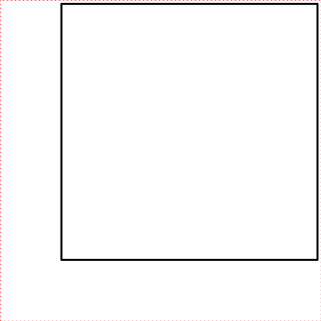### Line-width

The line width of the box can be modified easily with the parameter lwd = ...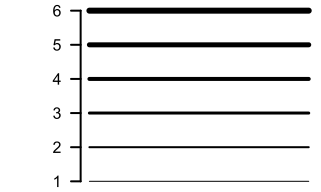This parameter can be applied to box or axis: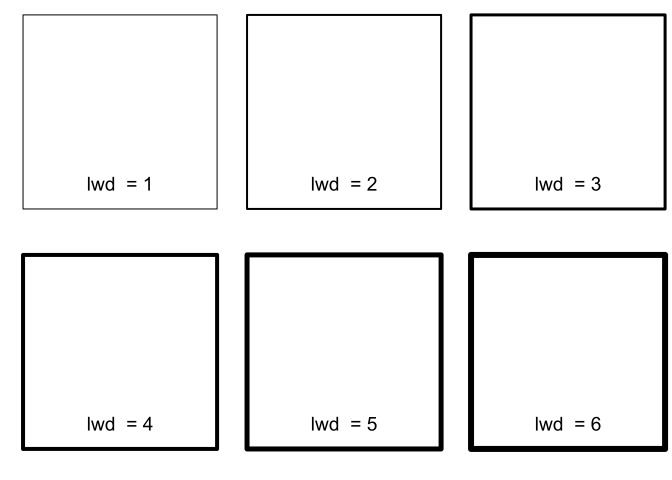### Line-type

There are six different line types. The line of the box can be modified easily with the parameter lty = ...: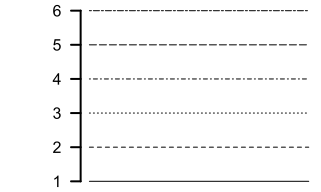This parameter can be easily applied to other graphical objects, such as box or axis: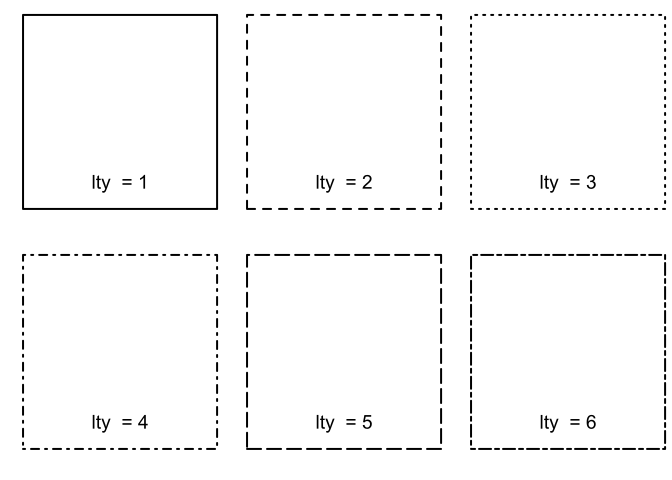### Line-color

The color of the line is easily defined with the parameter col= "..."):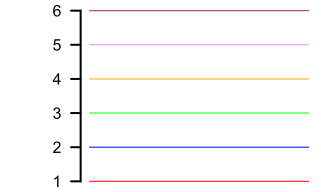This parameter can be applied to lines, axis or box: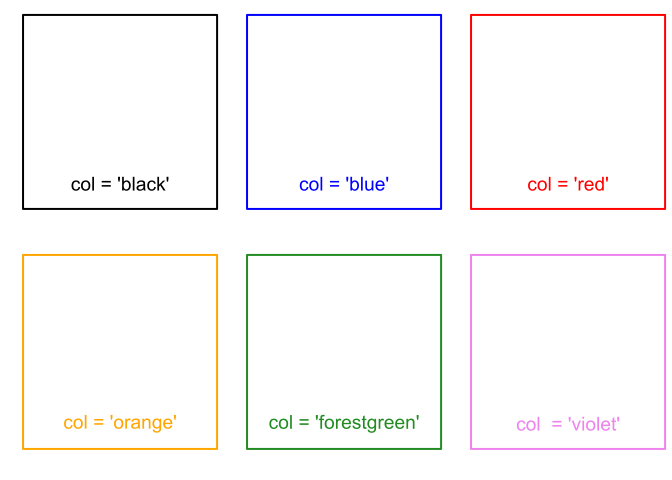## Forth step (a): draw points

Points can be added directly with the function plot() or matplot() or, in case a plot() function was already called, with the function points(). The graphical parameters for points symbols are the following:

Parameter Description
pch = 21 Set the symbol to be used (i.e. 21:25)
col = "red" Set the color border of the symbol
bg = "white" Set the color background for the symbol
cex = 1 Set the dimension for the symbol
lwd = 2 Set the line width for the symbol

It follows an example:

par(oma = c(0, 0, 0, 0))
par(mar = c(3, 3, 0, 0) + 0.2)
# DRAW AN EMPTY PLOT
plot(x,y1,
type = "n",
axes = F,
xlab = "",
ylab = "")
# DRAW BOX
box(which="plot", lwd = 2, lty = 1)
# DRAW POINTS
points(x,y1)
# DRAW A BOX AROUND THE DEVICE REGION
box(which="outer", col="red", lwd=1, lty=3)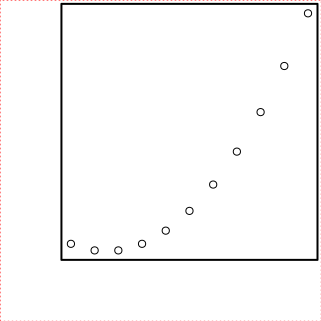Different points symbols can be selected by the parameter pch = ...: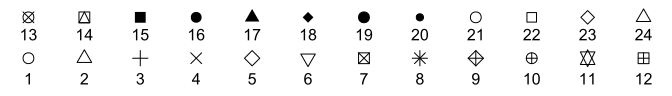Points can be drawn of any size (cex = ...) and color (col = "..."):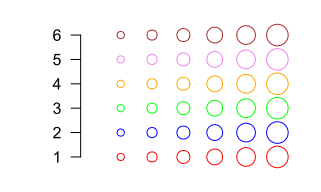Points from pch = 21:25 can be filled of any background color by the function bg = "..."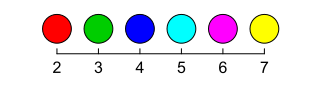and by any border color by the function col = "...":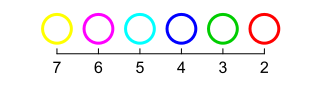Border thickness of symbols can be varied of any size by the function lwd = ...: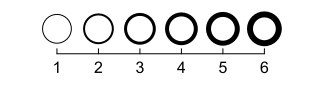## Forth step (b): draw lines

Once the empty plot is set and the box around the plot drawn, then, lines can be added with the function lines(). Some of the most common graphical parameters for lines are the following:

Parameter Description
lty = 1 Set six different line type (from 1 to 6)
lwd = 2 Set the line width
col = "red" Set the line color

It follows an example with points and lines together:

par(oma = c(0, 0, 0, 0))
par(mar = c(3, 3, 0, 0) + 0.2)
# DRAW AN EMPTY PLOT
plot(x,y1,
type = "n",  # EMPTY PLOT
axes = F,    # NO AXIS LINE
xlab = "",   # NO X-TICK LABELS
ylab = "")   # NO Y-TICK LABELS
# DRAW BOX
box(which="plot", lwd = 2, lty = 1)
# DRAW POINTS AND LINES
points(x,y1)
lines(x,y1)
# DRAW A BOX AROUND THE DEVICE REGION
box(which="outer", col="red", lwd=1, lty=3)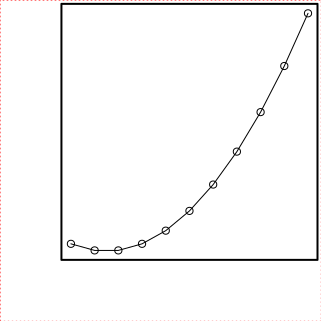As described previously, lines can be drawn with different color (col = ...) and thickness (lwd = ...):